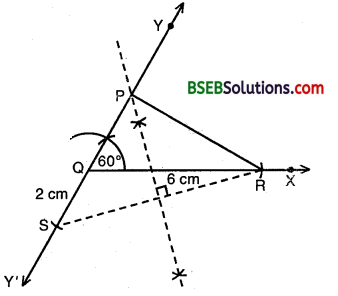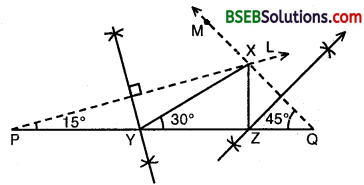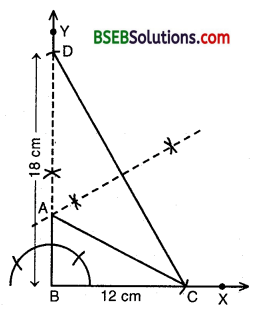# HSSlive: Plus One & Plus Two Notes & Solutions for Kerala State Board

## BSEB Class 9 Maths Chapter 11 Constructions Ex 11.2 Textbook Solutions PDF: Download Bihar Board STD 9th Maths Chapter 11 Constructions Ex 11.2 Book AnswersBSEB Class 9 Maths Chapter 11 Constructions Ex 11.2 Textbook Solutions PDF: Download Bihar Board STD 9th Maths Chapter 11 Constructions Ex 11.2 Book Answers

BSEB Class 9th Maths Chapter 11 Constructions Ex 11.2 Textbooks Solutions and answers for students are now available in pdf format. Bihar Board Class 9th Maths Chapter 11 Constructions Ex 11.2 Book answers and solutions are one of the most important study materials for any student. The Bihar Board Class 9th Maths Chapter 11 Constructions Ex 11.2 books are published by the Bihar Board Publishers. These Bihar Board Class 9th Maths Chapter 11 Constructions Ex 11.2 textbooks are prepared by a group of expert faculty members. Students can download these BSEB STD 9th Maths Chapter 11 Constructions Ex 11.2 book solutions pdf online from this page.

## Bihar Board Class 9th Maths Chapter 11 Constructions Ex 11.2 Books Solutions

 Board BSEB Materials Textbook Solutions/Guide Format DOC/PDF Class 9th Subject Maths Chapter 11 Constructions Ex 11.2 Chapters All Provider Hsslive

## How to download Bihar Board Class 9th Maths Chapter 11 Constructions Ex 11.2 Textbook Solutions Answers PDF Online?

2. Click on the Bihar Board Class 9th Maths Chapter 11 Constructions Ex 11.2 Answers.
3. Look for your Bihar Board STD 9th Maths Chapter 11 Constructions Ex 11.2 Textbooks PDF.
4. Now download or read the Bihar Board Class 9th Maths Chapter 11 Constructions Ex 11.2 Textbook Solutions for PDF Free.

Find below the list of all BSEB Class 9th Maths Chapter 11 Constructions Ex 11.2 Textbook Solutions for PDF’s for you to download and prepare for the upcoming exams:

## BSEB Bihar Board Class 9th Maths Solutions Chapter 11 Constructions Ex 11.2

Question 1.
Construct a triangle ABC in which BC = 7 cm, ∠B = 75° and AB + AC = 13 cm.
Solution:
Steps of Construction

1. Draw a ray BX and cut off a line segment BC = 7 cm.
2. Construct ∠XBY = 75°.3. From BY, cut off BD = 13 cm.
4. Join CD.
5. Draw the perpendicular bisect of CD, intersecting BA at A.
6. Join AC.

The triangle ABC thus obtained is the required triangle.

Question 2.
Construct a triangle ABC in which BC = 8 cm, ∠B = 45° and AB – AC = 3.5 cm.
Solution:
Steps of Construction :

1. Draw a ray BX and cut off a line segment BC = 8 cm from it.
2. Construct ∠YBC = 45°3. Cut off a line segment BD = 3.5 cm from BY.
4. Join CD.
5. Draw per-pendicular bisector of CD intersecting BY at a point A.
6. Join AC.

Then, ABC is the required triangle.

Question 3.
Construct a triangle PQR in which QR = 6 cm, ∠Q = 60° and PR – PQ = 2 cm.
Solution:
Steps of Construction :

1. Draw a ray QX and cut off a line segment QR = 6 cm from it.
2. Construct a ray QY making an angle of 60° with QR and produce YQ to form a line YQY’.3. Cut off a line segment QS = 2 cm from QY’.
4. Join RS.
5. Draw perpendicular bisector of RS intersecting QY at a point P.
6. Join PR.

Then, PQR is the required triangle.

Question 4.
Construct a triangle XYZ in which ∠Y = 30°, ∠Z = 90° and XY + YZ + ∠X = 11 cm.
Solution:
Steps of Construction :

1. Draw a line segment PQ = 11 cm.
2. At P, draw a ray PL such ∠LPQ = 12 x 30° = 15°3. At Q, draw ray QM such that ∠MQP = 12 x 90° = 45° intersecting PL at X.
4. Draw perpendicular bisectors of XP and XQ intersecting PQ in Y and Z respectively.

Then, ∆ XYZ is the required triangle.

Note : For clarity in figure, method of drawing angles of 15° and 45° have not been shown. Students should draw these angles with the help of ruler and compass only by the method as shown earlier.

Question 5.
Construct a right triangle whose base is 12 cm and sum of its hypotenuse and other side is 18 cm.
Solution:
Steps of Construction :

1. Draw a ray BX and cut off a line segment BC = 12 cm.
2. Construct ∠XBY = 90°.3. From By cut off a line segment BD = 18 cm.
4. Join CD.
5. Draw the per¬pendicular bisector of CD intersecting BD at A.
6. Join AC.

Then ABC is the required triangle.

## Bihar Board Class 9th Maths Chapter 11 Constructions Ex 11.2 Textbooks for Exam Preparations

Bihar Board Class 9th Maths Chapter 11 Constructions Ex 11.2 Textbook Solutions can be of great help in your Bihar Board Class 9th Maths Chapter 11 Constructions Ex 11.2 exam preparation. The BSEB STD 9th Maths Chapter 11 Constructions Ex 11.2 Textbooks study material, used with the English medium textbooks, can help you complete the entire Class 9th Maths Chapter 11 Constructions Ex 11.2 Books State Board syllabus with maximum efficiency.

## FAQs Regarding Bihar Board Class 9th Maths Chapter 11 Constructions Ex 11.2 Textbook Solutions

#### Can we get a Bihar Board Book PDF for all Classes?

Yes you can get Bihar Board Text Book PDF for all classes using the links provided in the above article.

## Important Terms

Bihar Board Class 9th Maths Chapter 11 Constructions Ex 11.2, BSEB Class 9th Maths Chapter 11 Constructions Ex 11.2 Textbooks, Bihar Board Class 9th Maths Chapter 11 Constructions Ex 11.2, Bihar Board Class 9th Maths Chapter 11 Constructions Ex 11.2 Textbook solutions, BSEB Class 9th Maths Chapter 11 Constructions Ex 11.2 Textbooks Solutions, Bihar Board STD 9th Maths Chapter 11 Constructions Ex 11.2, BSEB STD 9th Maths Chapter 11 Constructions Ex 11.2 Textbooks, Bihar Board STD 9th Maths Chapter 11 Constructions Ex 11.2, Bihar Board STD 9th Maths Chapter 11 Constructions Ex 11.2 Textbook solutions, BSEB STD 9th Maths Chapter 11 Constructions Ex 11.2 Textbooks Solutions,
Share: